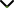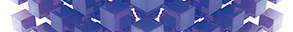# Fractional Mathematical Modelling and Optimal Control Problems of Differential Equations

Publishing date
01 Feb 2021
Status
Closed
25 Sep 2020

Guest Editors

1Curtin University of Technology, Perth, Australia

2Qufu Normal University, Qufu, China

3Yantai University, Yantai, China

This issue is now closed for submissions.
More articles will be published in the near future.

# Fractional Mathematical Modelling and Optimal Control Problems of Differential Equations

This issue is now closed for submissions.
More articles will be published in the near future.

## DescriptionMany dynamic systems and optimal control processes possess nonlocal character, and either the performance index or the differential equations governing the dynamics of the system involve fractional derivatives. Fractional models usually have higher accuracy than the traditional integer order differential equation models in depicting long term memory effects and viscoelastic processes.

Owing to the salient features and high accuracy of fractional mathematical modelling, it has attracted increasing research interest in the control community and mathematics field, and various fractional mathematical modelling and analysis methodologies have been developed, such as fractional calculus theory, control design of fractional problems, parameter estimation, and numerical methods. However, there are some unsolved problems and challenges in fractional mathematical modelling and optimal control problems, which deserve further investigation. New advancements in fractional models and control problems will promote the development of analysis theory, computational methods, and mathematical physics as well as their applications to differential and integral equations.

The aim of this Special Issue is to report and promote the latest achievements and recent developments in fractional mathematical modelling and optimal control. We welcome researchers to submit original research articles as well as review articles in relation to the following listed topics

Potential topics include but are not limited to the following:

• Nonlinear dynamic control problems with fractional derivative
• Fractional mathematics model with boundary value conditions
• Fractional mathematics model with initial value conditions
• Inequalities of fractional integrals and fractional derivatives
• Optimization with fractional differential equations
• Numerical analysis and algorithms for fractional differential equations
• Variational theory and fixed point theory in fractional calculus and their application
• Fractional space theory and application
• Operator theory in fractional calculusJournal metrics
Acceptance rate27%
Submission to final decision64 days
Acceptance to publication34 days
CiteScore1.800
Journal Citation Indicator0.400
Impact Factor1.305Author guidelinesEditorial boardDatabases and indexing

Article of the Year Award: Outstanding research contributions of 2020, as selected by our Chief Editors. Read the winning articles.